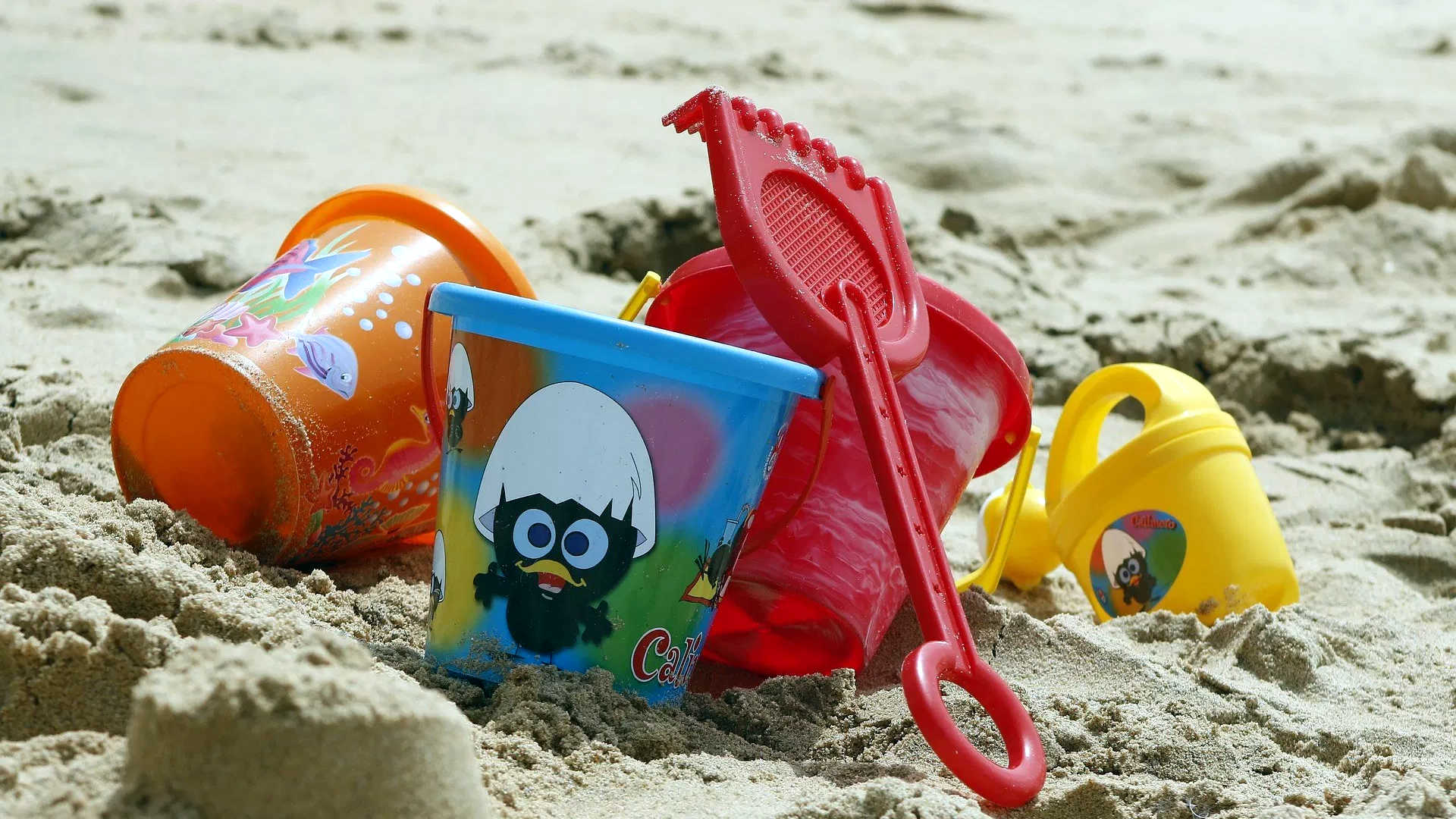# Bucketizing All The Things In C#

The Partition Problem is a very old conundrum in computer science. The usual question goes: given some buckets of some capacity, and some items of some size, how can I distribute the items by the buckets in an optimal manner?

The answer to this is more complex than it seems at first glance, as it depends on what you mean by some buckets and by optimal.

• Do you know in advance how many buckets you have? Or their size?
• Do you have to fill more buckets with fewer items or less buckets with more items?
• Do you allow bucket overflow or enforce capacity?
• Do you want the perfect distribution regardless of performance or is fast-and-furious good enough for you?

There are lots of ways of answering these questions and even more ways of implementing solutions for them, from simple greedy algorithms to more complex heuristics based ones.

Today I’ll get you started the basics for a simple and generic distribution algorithm based on a descending greedy approach, with a couple of variations:

• Fixed bucket count: Distribute all items up to available buckets. This is a common scenario and a good place to start.
• Fixed bucket size, uncapped bucket count: Prioritize filling up buckets up to their size, before adding new ones.
• Auto-capped bucket size, uncapped bucket count: Cap bucket size by the largest item, then behave as the previous method. This is useful when you want to adjust some work to the size of the longest task, so all tasks complete together as possible.

So let’s set this up.

## Bucket

First, we create a helper class to act as a bucket:

``````public class Bucket
{
public int TotalCost { get; set; } = 0;
public IList Items { get; } = new List();

private Func cost;

public Bucket(Func cost)
{
this.cost = cost;
}

{
TotalCost += cost(item);
}
}
``````

This class abstracts the bucket concept out of the algorithm and helps keep things simple. Note how the bucket accepts a cost function. This makes it simple to keep the current bucket cost updated without having to recalculate things all the time.

## Bucketizer

Now we add a Bucketizer class to hold the algorithms. You can also call it Potatoes, but I’ll leave that up to you.

``````public class Bucketizer
{
}
``````

## Bucketize By Bucket Count

So let’s say you know how many buckets you have available upfront. This variation will distribute any number of items over a fixed number of buckets. If you have fewer items than buckets, then the method returns only those buckets that got filled.

``````public Bucket[] BucketizeByBucketCount(IEnumerable items, Func cost, int maxBucketCount)
{
// validate
if (items == null) throw new ArgumentNullException(nameof(items));
if (cost == null) throw new ArgumentNullException(nameof(cost));
if (maxBucketCount  cost(x)).ToArray();

// check if there is anything to bucket
if (ordered.Length < 1)
{
// return the empty bucket array
return buckets.ToArray();
}

// how many items we have vs how many buckets
var needs = (ordered.Length < maxBucketCount ? ordered.Length : maxBucketCount);

// distribute items by bucket count
foreach (var item in ordered)
{
// can we still add buckets
}
}

// done
return buckets.ToArray();
}
``````

This simple algorithm takes all of the items, orders them by descending order, and then puts each item into the bucket with the most space available. However, it will create a new bucket first, up to the maximum number, before reusing existing buckets. The magic here is the descending order - this will give you very decent results most of the time while keeping the code easy to understand.

Note that the code above is not super fast - it has (buckets * items) max iterations plus the initial cost of ordering - but you can optimize it with a dictionary (keyed on current bucket weight) once you adjust it to your needs.

I’ll leave that exercise up to you.

## Bucketize By Bucket Size

So you don’t care about the number of buckets. Instead you want to fill up as few buckets as possible up a maximum bucket size. In that case, you can go with this:

``````public Bucket[] BucketizeByBucketSize(IEnumerable items, Func cost, int maxBucketSize)
{
// validate
if (items == null) throw new ArgumentNullException(nameof(items));
if (cost == null) throw new ArgumentNullException(nameof(cost));
if (maxBucketSize  cost(x)).ToArray();

// check if there is anything to bucket
if (ordered.Length  cost(i)).Max();

// bucketize by max bucket size using top item cost
return BucketizeByBucketSize(items, cost, top);
}
``````

All this code does is to discover the item with the highest cost, and the delegate the rest of the logic to the previous method. Nothing to it.

There are many other ways of bucketizing items and in far more optimal ways that the examples above - but I hope this gives you a head start.

Till next time.

Jorge Candeias's Picture